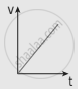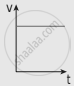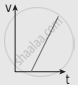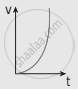Tamil Nadu Board of Secondary EducationHSC Science Class 11th

# The following graphs represent the velocity-time graph. Identify what kind of motion a particle undergoes in each graph. (a) (b) (c) (d) - Physics

Short Note

The following graphs represent the velocity-time graph. Identify what kind of motion a particle undergoes in each graph.

 (a)(b)(c)(d)#### Solution

(a) At all the points, the slope of the graph is constant.
∴ veca = constant

(b) No change in magnitude of the velocity with respect to time
∴ vecv = constant

(c) Slope of this graph is greater than graph (a) but constant
∴ veca = constant but greater than the graph (a)

(d) At each point-slope of the curve increases.
∴ veca is a variable and the object is in accelerated motion.

Concept: Motion Along One Dimension
Is there an error in this question or solution?
Chapter 2: Kinematics - Evaluation [Page 101]

Share